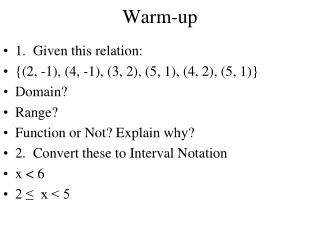DownloadDownload PresentationWarm-up

# Warm-up

Download Presentation## Warm-up

- - - - - - - - - - - - - - - - - - - - - - - - - - - E N D - - - - - - - - - - - - - - - - - - - - - - - - - - -
##### Presentation Transcript

1. Warm-up • 1. Given this relation: • {(2, -1), (4, -1), (3, 2), (5, 1), (4, 2), (5, 1)} • Domain? • Range? • Function or Not? Explain why? • 2. Convert these to Interval Notation • x < 6 • 2 ≤ x < 5

2. Warm-up • 1. Given this relation: • {(2, -1), (4, -1), (3, 2), (5, 1), (4, 2), (5, 2)} • Domain? {2,3,4,5} • Range? {-1,1,2} • Function or Not? NO, duplicated “x” values • 2. • x < 6 in interval notation (-∞, 6) • 2 ≤ x < 5 in interval notation [2, 5)

3. Continuous Functionsvs Discrete FunctionsDomain and Range Chapter 2 Section 2-1 Pages 72-81

4. Objectives • I can determine Domain and Range from a Continuous Graph • I can identify a discrete and continuous function

5. Important Vocabulary • Discrete Function • Continuous Function

6. Discrete Function • A function with ordered pairs that are just points and not connected.

7. Discrete Function

8. Continuous Functions?? • A function is continuous if it has an infinite domain and forms a smooth line or curve • Simply put: It has NO BREAKS!!! • You should be able to trace it with your pencil from left to right without picking up your pencil

9. y x 4 -4 The domain of the function y = f(x) is the set of values of x for which a corresponding value of y exists. Domain & Range The range of the function y = f(x) is the set of values of y which correspond to the values of x in the domain. Range Domain

10. Example: Domain & Range y 1 (–3, 0) x – 1 Example: Find the domain and range of the function f(x) = from its graph. Range Domain The domainis[–3,∞). The rangeis[0,∞).

11. Example 1

12. Example 2

13. Example 3

14. Homework • WS 1-5: Domain and Range